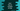# How to multiply strings in Dart## Dart multiply strings:

In this post, we will learn how to multiply a string for n number of times. We can use the multiplication operator * for that. Using this operator, we can concatenate the same string for n number of times.

In this post, we will learn how to use it with an example.

### Syntax of * operator:

* operator is defined as below:

``String operator * (int times)``

If we multiply the string for n times, i.e. str * n, it will produce the string n times concatenating with itself. It is like str + str +…n times

If we provide the n value as zero or negative, it will return an empty string.

### Example of * operator:

Let’s take a look at the below example:

``````void main() {
var given_str = "hello";

print(given_str * 4);
print(given_str * 0);
print(given_str * -1);
}``````

If you run this program, it will print the below output:

``````hellohellohellohello

``````

You can see that,

• The first print statement concatenate the same string 4 times.
• The second print statement returns empty string
• The third print statement returns empty string